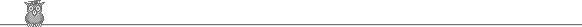### 27th USAMO 1998Problem A3

The reals x1, x2, ... , xn+1 satisfy 0 < xi < π/2 and ∑1n+1 tan(xi - π/4) ≥ n-1. Show that ∏1n+1 tan xi ≥ nn+1.

Solution

Put ti = tan(xi - π/4). Then (1 + ti)/(1 - ti) = tan(π/4 + xi - π/4) = tan xi. So we wish to show that ∏(1 + ti)/(1 - ti) ≥ nn+1.

The given inequality is equivalent to 1 + ti ≥ ∑j≠i (1 - tj). Using the AM/GM inequality, this implies that (1 + ti)/n >= ∏j≠i (1 - tj)1/n. Hence ∏ (1 + ti)/nn+1 ≥ ∏ij≠i (1 - tj)1/n = ∏ (1 - ti).